Test: Single Phase HW AC-DC - 2

# Test: Single Phase HW AC-DC - 2

Test Description

## 20 Questions MCQ Test Power Electronics | Test: Single Phase HW AC-DC - 2

Test: Single Phase HW AC-DC - 2 for Electrical Engineering (EE) 2022 is part of Power Electronics preparation. The Test: Single Phase HW AC-DC - 2 questions and answers have been prepared according to the Electrical Engineering (EE) exam syllabus.The Test: Single Phase HW AC-DC - 2 MCQs are made for Electrical Engineering (EE) 2022 Exam. Find important definitions, questions, notes, meanings, examples, exercises, MCQs and online tests for Test: Single Phase HW AC-DC - 2 below.
Solutions of Test: Single Phase HW AC-DC - 2 questions in English are available as part of our Power Electronics for Electrical Engineering (EE) & Test: Single Phase HW AC-DC - 2 solutions in Hindi for Power Electronics course. Download more important topics, notes, lectures and mock test series for Electrical Engineering (EE) Exam by signing up for free. Attempt Test: Single Phase HW AC-DC - 2 | 20 questions in 20 minutes | Mock test for Electrical Engineering (EE) preparation | Free important questions MCQ to study Power Electronics for Electrical Engineering (EE) Exam | Download free PDF with solutions
 1 Crore+ students have signed up on EduRev. Have you?
Test: Single Phase HW AC-DC - 2 - Question 1

### Choose the correct statement with respect to the below given circuit.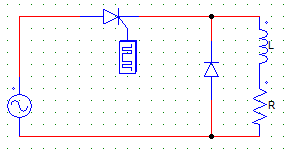Detailed Solution for Test: Single Phase HW AC-DC - 2 - Question 1

The voltage cannot be negative due to the FD (freewheeling diode or commutating diode so connected).

Test: Single Phase HW AC-DC - 2 - Question 2

### In the below given circuit, the FD (Freewheeling diode) is forward biased at ωt =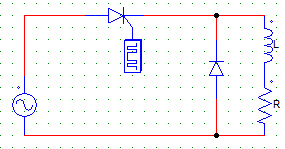Detailed Solution for Test: Single Phase HW AC-DC - 2 - Question 2

It is forward biased at π by the conducting SCR & the current starts to through the FD & Load.

Test: Single Phase HW AC-DC - 2 - Question 3

### In the below given circuit, when the commutating diode or FD is conducting than the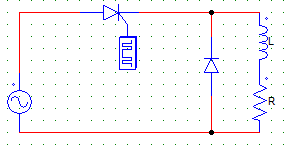Detailed Solution for Test: Single Phase HW AC-DC - 2 - Question 3

When the FD is forward biased at π by the conducting SCR & the current starts to through the FD & load.

Test: Single Phase HW AC-DC - 2 - Question 4

The output voltage waveform of the below given circuit would be the same that obtained from a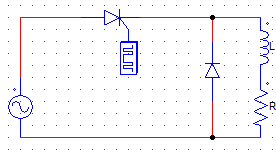Detailed Solution for Test: Single Phase HW AC-DC - 2 - Question 4

The wave from will be like a half wave diode rectifier circuit. Which is the same as that obtained from a half-wave R load circuit and the above given circuit.

Test: Single Phase HW AC-DC - 2 - Question 5

In a single-phase half-wave circuit with RL load and a freewheeling diode, the load voltage during the freewheeling period will be

Detailed Solution for Test: Single Phase HW AC-DC - 2 - Question 5

The FD short circuits the load and voltage across a short circuit would be = 0.

Test: Single Phase HW AC-DC - 2 - Question 6

In a single-phase half-wave circuit with RL load and a freewheeling diode, the freewheeling period is

Detailed Solution for Test: Single Phase HW AC-DC - 2 - Question 6

Freewheeling period is the one in which the FD diode conducts.

Test: Single Phase HW AC-DC - 2 - Question 7

A single-phase half wave rectifier with a FD is supplied by Vs = 240 V, AC with a load R = 10 Ω, L = 0.5 mH and a firing angle α = 30°. Find the average value of the load voltage.

Detailed Solution for Test: Single Phase HW AC-DC - 2 - Question 7

Vo = (Vm/2π) x (1+cosα)
Vm = √2Vs.

Test: Single Phase HW AC-DC - 2 - Question 8

A single-phase HW rectifier with a FD is supplied by Vs = 240 V, 50 Hz, AC with a load R = 10 Ω, L = 0.5 mH and a firing angle α = 30°. Find the average value of the load current.

Detailed Solution for Test: Single Phase HW AC-DC - 2 - Question 8

Vo = (Vm/2π) x (1+cosα)
Vm = √2Vs
Io = Vm/R (Due to the FD).

Test: Single Phase HW AC-DC - 2 - Question 9

A single phase half-wave controlled rectifier has 400 sin314t as the input voltage and R as the load. For a firing angle of 60°,the average output voltage is

Detailed Solution for Test: Single Phase HW AC-DC - 2 - Question 9

Vo = (Vm/2π) x (1+cosα) = 400/2π x (1+cos60) = 300/π.

Test: Single Phase HW AC-DC - 2 - Question 10

Choose the incorrect statement with respect to the use of FD in half-wave circuits.

Detailed Solution for Test: Single Phase HW AC-DC - 2 - Question 10

PIV is unaffected with the use of FD (freewheeling diodes).

Test: Single Phase HW AC-DC - 2 - Question 11

A single-phase half-wave rectifier with a FD is supplied by Vs = 240 V, 50 Hz, AC source with a load R = 10 Ω, L = 0.8 mH. The firing angle is so adjusted such that the output voltage obtained is 100 V. Find the firing angle.

Detailed Solution for Test: Single Phase HW AC-DC - 2 - Question 11

Vo = Vm/2π cos(firing angle).

Test: Single Phase HW AC-DC - 2 - Question 12

For the below shown circuit, a motor load (RLE) is connected to the SCR through supply Vs. The minimum value of the firing angle to turn on the SCR would be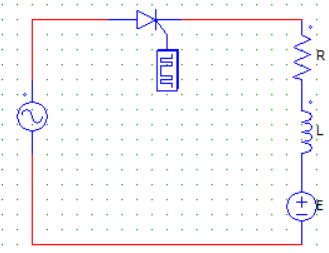Detailed Solution for Test: Single Phase HW AC-DC - 2 - Question 12

The firing angle should be such that the value of V exceeds E. So SCR turns on at excatlly Vm sinωt = E
Therefore ωt = min. angle = Sin-1(E/Vm).

Test: Single Phase HW AC-DC - 2 - Question 13

Choose the correct statement with respect to the below given circuit.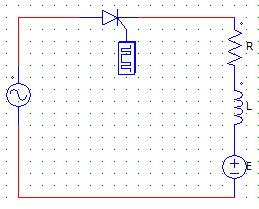Detailed Solution for Test: Single Phase HW AC-DC - 2 - Question 13

Even if no current is flowing through the load when the SCR is off, voltage = E will exists at the load terminals at all times.

Test: Single Phase HW AC-DC - 2 - Question 14

By using a freewheeling diode (FD) in a rectifier with RL load, the power consumed by the load

Detailed Solution for Test: Single Phase HW AC-DC - 2 - Question 14

The FD feeds inductor current again to the load.

Test: Single Phase HW AC-DC - 2 - Question 15

A 230 V, 50 Hz, one-pulse SCR controlled converter has extinction angle β = 210°. Find the circuit turn-off time

Detailed Solution for Test: Single Phase HW AC-DC - 2 - Question 15

t = 2π-β/ω
Where, π = 90°
ω = 2xπx50
β = 210°.

Test: Single Phase HW AC-DC - 2 - Question 16

A 230V, 50Hz, single-pulse SCR is feeding a RL load with α = 40° and β = 210°. Find the value of average output voltage

Detailed Solution for Test: Single Phase HW AC-DC - 2 - Question 16

Vo = Vm/2π x (cosα-cosβ)
Where Vm = √2Vs.

Test: Single Phase HW AC-DC - 2 - Question 17

A single-pulse transformer with secondary voltage of 230 V, 50 Hz, delivers power to bulb of R = 10 Ω through a half-wave controlled rectifier circuit. For α = 60°, find the average current in the bulb

Detailed Solution for Test: Single Phase HW AC-DC - 2 - Question 17

First find the average voltage, than Io = Vo/R
Vo = (Vm/2π) x (1+cosα).

Test: Single Phase HW AC-DC - 2 - Question 18

A single-pulse transformer with secondary voltage of 230 V, 50 Hz, delivers power to bulb of R = 10 Ω through a half-wave controlled rectifier circuit. For α = 60° and output AC power of 2127 Watts, find the rectification efficiency

Detailed Solution for Test: Single Phase HW AC-DC - 2 - Question 18

Vo = (Vm/2π) x (1+cosα) = 77.64 V
Pdc = Vo2xR = 602.8 W
Rectification efficiency = Pdc/Pac = 28.32 %.

Test: Single Phase HW AC-DC - 2 - Question 19

Find the PIV of the SCR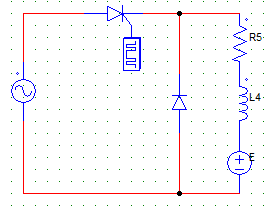Detailed Solution for Test: Single Phase HW AC-DC - 2 - Question 19

The maximum negative voltage faced by the SCR is –Vm which is nothing but √2Vs.

Test: Single Phase HW AC-DC - 2 - Question 20

An SCR rectifier circuit is designed such that the average output voltage is 77.64 V & RMS value of output voltage is 145.873 V. Find the voltage ripple factor.

Detailed Solution for Test: Single Phase HW AC-DC - 2 - Question 20

FF = 145.873/77.64 = 1.879
VRF = √(FF2-1) = 1.5908.

## Power Electronics

5 videos|13 docs|49 tests
 Use Code STAYHOME200 and get INR 200 additional OFF Use Coupon Code
Information about Test: Single Phase HW AC-DC - 2 Page
In this test you can find the Exam questions for Test: Single Phase HW AC-DC - 2 solved & explained in the simplest way possible. Besides giving Questions and answers for Test: Single Phase HW AC-DC - 2, EduRev gives you an ample number of Online tests for practice

## Power Electronics

5 videos|13 docs|49 tests